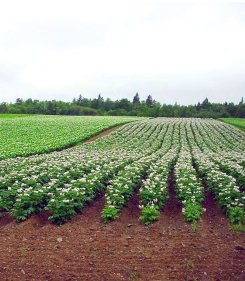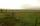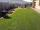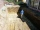# Garden

Trapezoid garden has parallel sides 19 m and 24 m. Its area is 193.5 square meters. What is the width of the garden?

x =  9 m

### Step-by-step explanation:We will be pleased if You send us any improvements to this math problem. Thank you!#### You need to know the following knowledge to solve this word math problem:

We encourage you to watch this tutorial video on this math problem:

## Related math problems and questions:

• Rectangle fieldThe field has a shape of a rectangle having a length of 119 m and a width of 19 m. , How many meters have to shorten its length and increase its width to maintain its area and circumference increased by 24 m?
• GardensThe area of the square garden is 3/4 of the area of the triangular garden with sides of 80 m, 50 m, 50 m. How many meters of the fence do we need to fence a square garden?
• The gardenThe garden has the shape of a rectangular trapezium. The bases have lengths of 27 meters and 36 meters, the trapezoid's height is 12 meters. Calculate how much fence will cost this garden if one meter costs 1.5 €?
• GardenThe garden has two opposite parallel fences. Their distance is 33.1 m. Lengths in these two fences are 75.5 meters and 49.4 meters. Calculate the area of this garden.
• Extending square gardenMrs. Petrová's garden had the shape of a square with a side length of 15 m. After its enlargement by 64 m2 (square), it had the shape of a square again. How many meters has the length of each side of the garden been extended?
• The gardenThe garden has the shape of an isosceles trapezoid whose bases are 64 m and 24 m long, the height is 25 m. On what area of the garden is it possible to grow vegetables if a fifth of the area is occupied by a cottage, lawn and path?
• GardenThe garden has the shape of a rectangle measuring 19m20cm and 21m60cm. Mr. Novák will fence it. It wants the distance between adjacent pillars to be at least two meters and a maximum of three meters. He would also like the distances between the adjacent p
• Trapezoidtrapezoid ABCD a = 35 m, b=28 m c = 11 m and d = 14 m. How to calculate its area?
• FenceThe square garden has an area of 537 m2. How many meters is netting necessary to fence the garden?
• GardenThe rectangular garden has dimensions of 27 m and 30 m. Peter and Katka split it in a ratio of 4:5. How many square meters did Katkin measure part of the garden?
• Horses playgroundThe fence for the horses has the shape of a rectangular trapezoid with an area of 400 m2, the base lengths should be 31 m and 19 m. How many meters of boards will they need to fence it if the boards are stacked in 5 rows?
• GardenRectangular garden has a length 99 m and width 84 m. Calculate how many m2 will decrease its area if land by ornamental fence with width 30 cm.
• The trenchCalculate how many cubic meters of soil needs to be removed from the excavation in the shape of an isosceles trapezoid, the top width is 3 meters, the lower width is 1.8 m, the depth of the excavation is 1 m, and the length is 20 m.
• Children playgroundThe playground has a trapezoid shape, and the parallel sides have a length of 36 m and 21 m. The remaining two sides are 14 m long and 16 m long. Find the size of the inner trapezoid angles.
• The schoolyardThe schoolyard had the shape of a square with an 11m side. The yard has been enlarged by 75 m2 and has a square shape again. How many meters was each side of the yard enlarged?
• GardenThe area of a square garden is 6/4 of triangle garden with sides 56 m, 35 m, and 35 m. How many meters of fencing need to fence a square garden?
• Mr. ZucchiniMr. Zucchini had a rectangular garden whose perimeter is 28 meters. The garden's content area filled just four square beds, whose dimensions in meters are expressed in whole numbers. Determine what size could have a garden. Find all the possibilities and